# ISEE Middle Level Math : How to find percentage

## Example Questions

### Example Question #81 : Percentage

Find the decimal equivalent of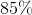.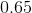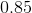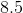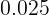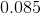Explanation:

To solve, divide by one hundred: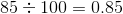Answer:### Example Question #82 : Percentage

Find the decimal equivalent of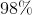.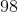None of these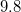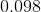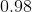Explanation:

To solve, divide by one hundred: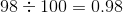Answer:### Example Question #83 : Percentage

Find the decimal equivalent of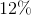.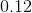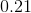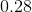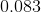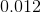Explanation:

To solve, divide by one hundred: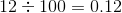Answer:### Example Question #84 : Percentage

Find the decimal equivalent of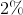.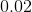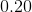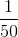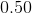Explanation:

To solve, divide by one hundred: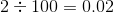Answer:### Example Question #85 : Percentage

What is the equivalent of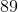%.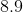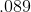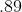Explanation:

To solve, divide: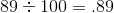Answer:### Example Question #86 : Percentage

What is the equivalent of%.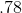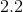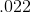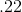Explanation:

To solve, divide: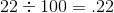Answer:### Example Question #87 : Percentage

What is the equivalent of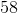%.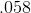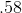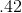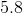Explanation:

To solve, divide: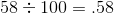Answer:### Example Question #88 : Percentage

What is the equivalent of%.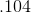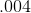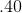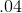Explanation:

To solve, divide: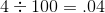Answer:### Example Question #89 : Percentage

What is the equivalent of%?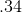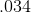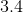Explanation:

To solve, divide: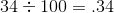Answer:### Example Question #90 : Percentage

What is the equivalent of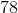%?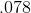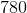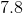To solve, divide: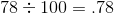Answer: